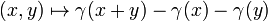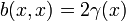## Definition

The universal quadratic functor (sometimes called Whitehead's universal quadratic functor) is a functor from abelian groups to abelian groups defined as follow. For an abelian group$G$ it outputs a group$\Gamma(G)$ given as the quotient of a free group on all the symbols$\gamma(x), x \in G$ by the following types of relations:

•$\gamma(0)= 0$ (this condition is redundant)
•$\gamma(-x) = \gamma(x) \ \forall \ x \in G$
•$\gamma(x) + \gamma(y) + \gamma(z) + \gamma(x + y + z) = \gamma(y + z) + \gamma(x + y) + \gamma(x + z) \ \forall x,y,z \in G$.

Note that the above set of relations is equivalent to the following pair of assumptions:

• The mapping$x \mapsto \gamma(x)$ is homogeneous of degree two:$\gamma(nx) = n^2 \gamma(x)$ for all$n \in \mathbb{Z}$
• The mapping$(x,y) \mapsto \gamma(x + y) - \gamma(x) - \gamma(y)$ is a bihomomorphism, i.e., it is additive in each coordinate.

### Equivalence of definitions

Further information: equivalence of definitions of universal quadratic functor

## Facts

• The exponent of$\gamma(x)$ in$\Gamma(G)$ divides twice the exponent of$x$ in$G$. This follows from noting that the bilinear form$b(x,y) = \gamma(x + y) - \gamma(x) - \gamma(y)$ also satisfies$b(x,-x) = -2\gamma(x)$ so$b(x,x) = 2\gamma(x)$, and the exponent of$b(x,x)$ divides the exponent of$x$ due to biadditivity.
• Formula for universal quadratic functor of direct product

## Particular cases

Starting group$G$ Universal quadratic functor$\Gamma(G)$ Comments
finite cyclic group of odd order$n$, i.e.,$\mathbb{Z}_n$$\mathbb{Z}_n$ Intuitively, this is saying that if$x$ is defined mod$n$ for$n$ odd, then$x^2$ is defined mod$n$ but we cannot do better in general
finite cyclic group of even order$n$, i.e.,$\mathbb{Z}_n$$\mathbb{Z}_{2n}$ Intuitively, this is saying that if$x$ is defined mod$n$ for$n$ even, then$x^2$ is defined mod$2n$ but we cannot do better in general
group of integers$\mathbb{Z}$$\mathbb{Z}$ we can think of the generator for$\Gamma(G)$ as the squaring map in the ring of integers